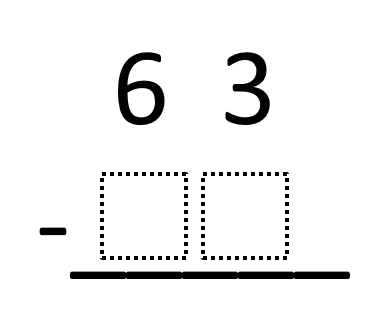Home > Grade 4 > Subtraction with Regrouping

# Subtraction with Regrouping

Directions: Fill in the boxes so that you would need to regroup when you subtract. Make sure that your number is less than 63.

Extension: Explain why you need to regroup using your number.### Hint

What number can you NOT subtract from 3?

The student puts in a number less than 63 and does not contain a 0, 1, 2, or 3 in the ones place.

Source: Chase Orton

## Multiplying Differences

Directions: Using the digits 1 to 9, at most one time each, fill in the …

1.Awesome problem. However, the hint just perpetuates the misconception that you can’t subtract a bigger number from a smaller number. I see this misconception follow some students even through middle school. It’s ok to tell students that later they will be subtracting 7 from 3 and getting -4. This is not a foreign concept to students. Think of temperature or games where you lose points and can drop below 0.

•That’s a great point Michael, and a really bad oversight on my part. I’m a big proponent of not teaching students rules that expire (ie, to multiply by 10, just add a zero) and rules limit conceptual understanding and fluency.

What might be a better hint we could put in there instead?

•When you have the updated hint, we’ll make the change. Certainly challenging to get the wording just right.

2.Maybe the hint should not be worded as subtracting a number, but rather subtracting a digit. For example: What digit cannot be subtracted from 3 without regrouping? A larger digit in the ones place requires a student to regroup since this is a 2-digit by 2-digit subtraction problem. What do you all think?

•